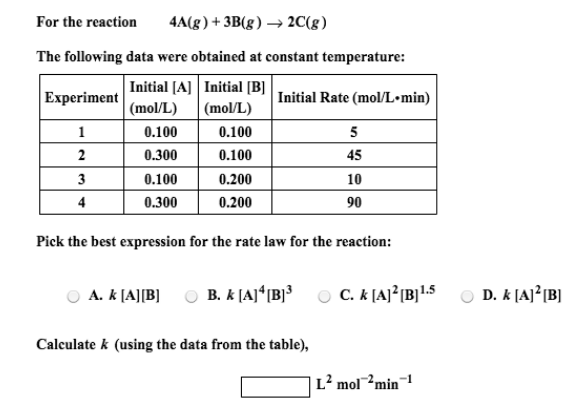# Problem: For the reaction          4A(g) + 3B(g) → 2C(g) The following data were obtained at constant temperature: Pick the best expression for the rate law for the reaction: Calculate k (using the data from the table),

###### FREE Expert Solution
80% (186 ratings)###### Problem Details

For the reaction          4A(g) + 3B(g) → 2C(g)

The following data were obtained at constant temperature:

Pick the best expression for the rate law for the reaction:

Calculate k (using the data from the table),